Structural Bioinformatics Library Template C++ / Python API for developping structural bioinformatics applications.
T_Space_filling_model_coarse_graining_outer_cover< AlphaComplex3 > Class Template Reference

Engine computing the outer cover of a set of balls from its inner cover. More...

#include <Space_filling_model_coarse_graining_outer_cover.hpp>

## Classes

struct  Apollonius_cell
Representation of a cell in the Apollonius diagram. More...

struct  Larger_distance_priority
Functor comparing the distance of two points to the center of an input sphere. More...

struct  Larger_increment_priority
Sort the Apollonius cells by decreasing volume constribution of their associated sphere. More...

## Public Types

typedef T_Space_filling_model_coarse_graining_outer_cover< AlphaComplex3 > Self

typedef AlphaComplex3::Geom_traits::Kernel K
3D geometric kernel defining the objects used in the alpha-complex. More...

typedef K::FT FT
Number type used for representing the objects in the alpha-complex. More...

typedef K::Point_3 Point_3
Center of a sphere in the inner approximation. More...

typedef K::Sphere_3 Sphere_3
Representation of a sphere in the inner approximation. More...

## Constructors

T_Space_filling_model_coarse_graining_outer_cover (void)
Default constructor. More...

## Functor

template<class SphereIterator , class PointIterator , class OutputIterator >
OutputIterator operator() (SphereIterator begin_spheres, SphereIterator end_spheres, PointIterator begin_points, PointIterator end_points, OutputIterator out) const
Create an outer cover of the input points whose balls are centered on the input spheres, using the Apollonius diagram. More...

## Detailed Description

### template<class AlphaComplex3> class T_Space_filling_model_coarse_graining_outer_cover< AlphaComplex3 >

Engine computing the outer cover of a set of balls from its inner cover.

Let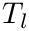be an input family of 3D balls,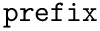the domain of their union, and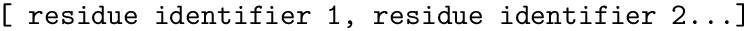the boundary of the domain. We make the following assumptions:

•has been meshed – see package Union_of_balls_mesh_3, resulting on a point cloud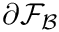,
• for some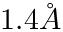, the one-sided Hausdorff distance between the boundary and the samples satisfies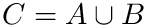.

To solve the outer problem, we enlarge the balls from the set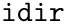, so as to cover the portion ofsticking out from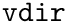.

Let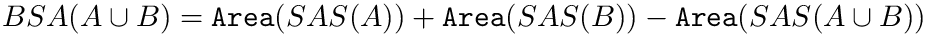be a point set initially consisting of the points from the sampled boundary not covered by.

Let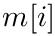be the set of balls to be expanded, initialized as the subset of balls from the selection contributing to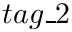.

For a given ball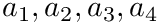, we proceed in two stages. First, the point in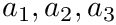maximizing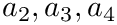is computed.

To account for the discretization, the corresponding additive distance is increased by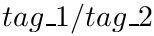.

Second, the ball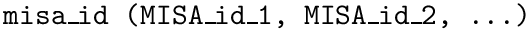is removed from, and all points in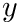are removed from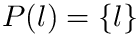. The process is iterated until exhaustion of.

Note that the previous algorithm does not require computing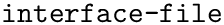, since the assignment of a point to its Apollonius Voronoi cell only requires computing its additive distances to all balls in.

Template Parameters
 AlphaComplex3 Model of an alpha-complex used for computing the volume of the different covers.

## ◆ FT

 typedef K::FT FT

Number type used for representing the objects in the alpha-complex.

## ◆ K

 typedef AlphaComplex3::Geom_traits::Kernel K

3D geometric kernel defining the objects used in the alpha-complex.

## ◆ Point_3

 typedef K::Point_3 Point_3

Center of a sphere in the inner approximation.

## ◆ Self

 typedef T_Space_filling_model_coarse_graining_outer_cover Self

## ◆ Sphere_3

 typedef K::Sphere_3 Sphere_3

Representation of a sphere in the inner approximation.

## ◆ T_Space_filling_model_coarse_graining_outer_cover()

 T_Space_filling_model_coarse_graining_outer_cover ( void )
inline

Default constructor.

## ◆ operator()()

 OutputIterator operator() ( SphereIterator begin_spheres, SphereIterator end_spheres, PointIterator begin_points, PointIterator end_points, OutputIterator out ) const
inline

Create an outer cover of the input points whose balls are centered on the input spheres, using the Apollonius diagram.